# Short Answer Questions: Real Numbers - 1 Notes | Study Mathematics (Maths) Class 10 - Class 10

## Class 10: Short Answer Questions: Real Numbers - 1 Notes | Study Mathematics (Maths) Class 10 - Class 10

The document Short Answer Questions: Real Numbers - 1 Notes | Study Mathematics (Maths) Class 10 - Class 10 is a part of the Class 10 Course Mathematics (Maths) Class 10.
All you need of Class 10 at this link: Class 10

Q 1. Consider the number 12n, where n is a natural number. Check whether there is any value of n ∈ N for which 12n ends with the digit zero or 5.

∵ Any number ending with the digit zero is always divisible by 5.
∴  If 12n ends with the digit zero, then it must be divisible by 5.
⇒ Prime factorisation of 12n must contain a prime factor 5.
Now,
12 = 2 × 2 × 3 = 22 × 3
⇒ (12)n = (22 × 3)n = 22n × 3n
∴  The prime factorisation of 12n does not contain the prime factor 5.
⇒ There is no value of n N such that 12n ends with the digit zero.

Q 2. If ‘p’ is prime, prove that √p is irrational.

Let p be rational in the simplest form a/b, where p is prime.
∴ a and b are integers having no common factor other than 1 and b ≠ 0.
⇒ Now, p = a/b
⇒ Squaring both sides, we have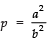⇒ pb= a2      ...(1)
Since pb2 is divisible by p, a2 is also divisible by p.
⇒ a is also divisible by p    ...(2)
Let a = pc for some integer c.
⇒ Substituting a = pc in (1), we have
pb2 = (pc)2
⇒ pb= p2c2
⇒ b2  = pc2
pc2 is divisible by p,
∴ b2 is divisible by p
⇒b is divisible by p    ...(3)
From (2) and (3),
⇒ p is a common factor of ‘a’ and ‘b’. But this contradicts our assumption that a and b are co-prime.
∴ Our assumption that p is rational is wrong. Thus, p is irrational if p is prime.

Q 3. Find the HCF of 18 and 24 using prime factorisation.

Using factor tree method, we have: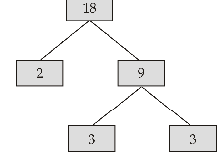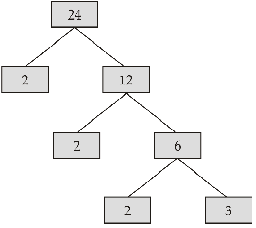∴ 18 = 2 × 3 × 3 = 2 × 32
24 = 2 × 2 × 2 × 3 = 23 × 3
HCF = Product of common prime factors with lowest powers.
⇒ HCF (18, 24) = 3 × 2  = 6

Q 4. Find the LCM of 10, 30 and 120.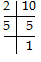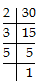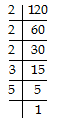∴ 10 = 2 × 5 = 21 × 51
30 = 2 × 3 × 5 = 21 × 31 × 51
120 = 2 × 2 × 2 × 3 × 5 = 23 × 31 × 51
LCM = Product of each prime factor with highest powers
⇒ LCM of 10, 30 and 120 = 23 × 3 × 5 = 120.

Q 5. Express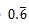as a rational number in the simplest form.

Let x= 0.666 .....    ...(1)
∴ 10x = 0.666 ..... × 10
= 6.666 .......(2)
Subtracting (1) from (2), we have:
10x - x = 6.666 ..... - 0.666 .....
⇒ 9x = 6
⇒ x = 6/9 = 2/3
Thus,= 2/3

Q 6. Express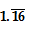as a rational number in the simplest form.

Let    x= 1.161616 ........(1)
∴ 100x = (1.161616 .....) × 100
⇒ 100x = 116.161616 ........(2)
Subtracting (1) from (2), we have:
100x - x = [116.161616 .....] – [1.161616 .....]
⇒ 99x = 115
⇒ x = 115/99
Thus,=115/99

Q 7. Use Euclid's division algorithm to find HCF of 870 and 225.

We have 870 = 3 × 225 + 195
225 = 1 × 195 + 30
195 =  6 × 30 + 15
30 =  2 × 15 + 0
∴ HCF (870, 225) is 15.

Note:
LCM of two numbers = Product of the numbers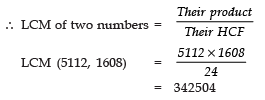Q 8. Find the LCM and HCF of 1296 and 5040 by prime factorisation method: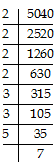and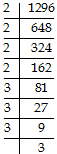∴ 5040 = 2 × 2 × 2 × 2 × 3 × 3 × 5 × 7
= 24 × 32 × 5 × 7  and
1296 =  2 × 2 × 2 × 2 × 3 × 3 × 3 × 3
= 24 × 34
∴ LCM = Product of each prime factor with highest powers
=  24 × 34 × 5 × 7
= 16 × 81 × 5 × 7 = 45360
HCF = Product of common prime factors with lowest powers
= 24 × 32
= 16 × 9 = 144

Q 9. Prove that √3 is irrational.

Let 3 be rational in the simplest form of P/q.
i.e., p and q are integers having no common factor other than 1 and q ≠ 0.
Now,   3 = p/q
Squaring both sides, we have
(√3)2 = (p/q)2
⇒ 3 = p2/q2
⇒ 3q2 = p............(1)
Since 3q2 is divisible by 3
∴ p2 is also divisible by 3
⇒ p is divisible by 3 ..........(2)
Let p = 3c for some integer ‘c’.
Substituting p = 3c in (1), we have:
3q = (3c)2
⇒ 3q2 = 9c2
⇒ q2  = 3c2
3cis divisible by 3
∴ q2 is divisible by 3
⇒ q is divisible by 3   ...(3)
From (2) and (3)
3 is a common factor of ‘p’ and ‘q’. But this contradicts our assumption that p and q are having no common factor other than 1.
∴ Our assumption that 3 is rational is wrong.
Thus, 3 is an irrational.

Q 10. Show that  3√2 is irrational.

Let 3√2 be a rational number
∴ p/q = 3√2 where p and q are prime to each other and q ≠ 0.
∴ p/3q = √2   ...(1)
Since, p is integer and 3q is also integer (3q≠ 0).
∴ p/3q is a rational number.
From (1), √2 is an integer
But this contradicts the fact that √2 is irrational.
⇒ 3√2  is irrational.

Q 11. Show that 2 - √3 is an irrational number.

Let  2 - √3 is rational.
∴ It can be expressed as p/q where p and q are integers (prime to each other) such that q ≠ 0.
∴  2 - √3   =  p/q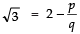...(1)

∵ p is an integer}
∴ q is an integer}
⇒ p/q is a rational number.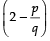is a rational number.   ...(2)
From (1) and (2), √3 is a rational number. This contradicts the fact that √3 is an irrational number.
∴ Our assumption that (2 -√3) is a rational number is not correct. Thus, (2 - √3) is irrational.

Q 12. Using Euclid’s division algorithm, find the HCF of 56, 88 and 404.

Using Euclid’s division algorithm to 88 and 56, we have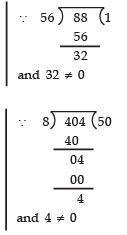3q2 = (3c)2
3q2 = (3c)2
88 = 56 × 1 + 32
56 = 32 × 1 + 24
32 = 24 × 1 + 8
24 = 8 × 3 + 0
∴ HCF of 88 and 56 is 8
Again, applying Euclid’s division algorithm to 8 and 404, we have:
404 = 8 × 504 + 4
8 = 4 × 2 + 0
∴ HCF of 404 and 8 is 4
Thus, HCF of 88, 56 and 404 is 4.

Q 13. Express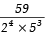in the decimal form.

We have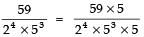[multiplying and dividing by 5]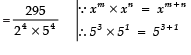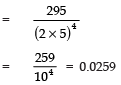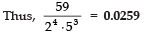Q 14. Express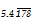in the p/q form.

Let    x    =or x = 5.4178178178 .....
∴ 10x  = 54.178178178 .....    ...(1)
Also  1000 (10x)=    54178.178178178 .....
⇒ 10000x    =    54178.178178178 .....    ...(2)
Subtracting (1) from (2), we have:
10000x - 10x
= [54178.178178 .....] - [54.178178 .....]
⇒ 9990x = 54124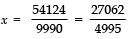Thus,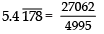Q 15. State whether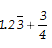is a rational number or not.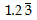= 1.23333 ..... is a non-terminating repeating decimal.is a rational number.
3/4 is in the form of p/q, where q ≠ 0  [Here 4 ≠ 0]
∴ 3/4 is a rational number.
Since the sum of two rational numbers is a rational number.
Therefore,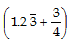is a rational number.

Q 16. The LCM of two numbers is 45 times their HCF. If one of the numbers is 225 and sum of their LCM and HCF is 1150, find the other number.

One of the numbers = 225
Let the other number = x
Also LCM = 45 (HCF)                 ...(1)
And LCM + HCF =1150
⇒ (45 HCF) + HCF = 1150
⇒ 46 HCF = 1150
⇒ HCF = 1150/46 = 25
From (1),
LCM = 45 × 25
∴ LCM × HCF = (45 × 25) × 25
Now,  LCM × HCF = Product of the numbers
∴  x × 225 = (45 × 25) × 25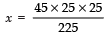= 125
Thus, the required number is 125.

Q 17. Three different containers contain 496 litres, 806 litres and 713 litres of a mixture. What is the capacity of the biggest container that can measure all the different quantities exactly?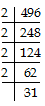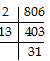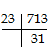∴ 496 = 2 × 2 × 2 × 2 × 31
= 24 × 31
806 = 2 × 13 × 31
713 = 23 × 31
⇒ HCF = 31
Thus, the required measure of the biggest container = 31 litres.

Q 18. Prove that 3+√2 is an irrational number.

Let 3 + √2 is a rational number.
∴ 3 + √2  = a/b such that ‘a’ and ‘b’ are co-prime integers and b ≠ 0.
We have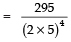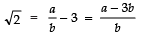Since a and b are integers,
∴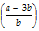is rational.
⇒√2 is a rational. This contradicts the fact that √2 is irrational.
∴ Our assumption that 3 + √2 is rational is not correct.
⇒ (3 + √2) is an irrational number.

The document Short Answer Questions: Real Numbers - 1 Notes | Study Mathematics (Maths) Class 10 - Class 10 is a part of the Class 10 Course Mathematics (Maths) Class 10.
All you need of Class 10 at this link: Class 10Use Code STAYHOME200 and get INR 200 additional OFF

## Mathematics (Maths) Class 10

58 videos|354 docs|138 tests

Track your progress, build streaks, highlight & save important lessons and more!

,

,

,

,

,

,

,

,

,

,

,

,

,

,

,

,

,

,

,

,

,

;